# Computer Science : Common Data Structures

## Example Questions

### Example Question #1 : Arrays

Consider the following code:

public static void main(String[] args) {

int[] vec = {8,-2,4,5,-8};

foo(vec);

}

private static void foo(int[] x) {

for(int i = 0; i < x.length; i++) {

int y = Math.abs(x[i]);

for(int j = 0; j < y; j++) {

System.out.print(x[i] + " ");

}

System.out.println();

}

}

Which of the following represents a possible output for the program above?

8 8 8 8 8 8 8 8

4 4 4 4

5 5 5 5 5

37

64

-4

16

25

-64

8 8 8 8 8 8 8 8

-2 -2

4 4 4 4

5 5 5 5 5

-8 -8 -8 -8 -8 -8 -8 -8

64

4

16

25

64

8 8 8 8 8 8 8 8

-2 -2

4 4 4 4

5 5 5 5 5

-8 -8 -8 -8 -8 -8 -8 -8

Explanation:

In this code's loop, notice that it takes the absolute value of each element.  This is done on the line:

int y = Math.abs(x[i]);

This value is then used for the second loop, which goes for y times, each time outputting the value of the given member of the original array—but now with its particular sign value.  Thus, even numbers likewill be output multiple times (i.e. 8).  This is done line by line for each member of the parameter array.

### Example Question #31 : Common Data Structures

Consider the following code:

public static void main(String[] args) {

double[][] matrix = {{1,6,7},{1,4,5}};

graphics(matrix);

}

private static double graphics(double[][] x) {

double r = 0;

for(int i = 0; i < x.length; i++) {

for(int j = 0; j < x[i].length; j++) {

r += x[i][j] * (i + 1);

}

}

return r;

}

What is the return value for graphics in the code below:

double[][] matrix = {{1,6,7},{1,4,5}};

graphics(matrix);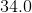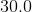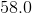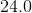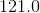Explanation:

The graphics method takes the 2D array matrix and then runs through each element.  This is the point of the double for loop in the method itself.  Notice that for each element it does several things.  First of all, it is clearly accumulating a value into the variable r.  Now, for each element, you are adding:

x[i][j] (the current value in the 2D array iteration)

TIMES

(i + 1), or, the current row number.  Thus, for the data given you are doing the following:

1* 1 + 1 * 6 + 1 * 7 + 2 * 1 + 2 * 4 + 2 * 5 = 34

### Example Question #91 : Standard Data Structures

For the following question, consider the following code:

public static void main(String[] args)

{

double[] vec = {4,8,10,18};

System.out.println(fun(vec));

privatestaticdouble fun(double[] x)

{

double p = 0;

for(int i = x.length-1; i > -1; i--)

{

p += x[i];

}

return p / x.length;

}

Which of the following is a possible output for this program?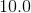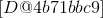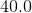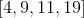Explanation:

First of all, you can eliminate the following two options very quickly, as the fun method returns a double value:

[D@4b71bbc9]

[4,9,11,19]

These are trying to trick you into thinking that it returns an array.  (The first value is really what would be the output, since there is no toString defined for the double array by default.)

Now, in fun, the loop simply runs through the values in the array, summing those up.  Notice, it runs "backwards" through the array, from the end to the beginning.  It then divides by the length of the array, giving you the average of the values in the array.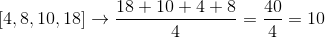### Example Question #1 : Arrays

Consider the following code:

String s = "I read logic for fun!";

boolean[] b = {true,false,true,false,false,true,true,false,true,false,true,false,false,true,false,true,true,false,true,false,false};

for(int i = 0; i < s.length(); i++)        {

char c = s.charAt(i);

if(!b[i]) {

c = Character.toUpperCase(c);

} else {

c = Character.toLowerCase(c);

}

System.out.print(c);

}

What is the output for this function code?

The code will throw an exception.

Explanation:

This code is using what are called parallel arrays.  The boolean array has one value for each character in the String s.  Notice the logic in the loop.  There is a condition:

if(!b[i]) {

c = Character.toUpperCase(c);

} else {

c = Character.toLowerCase(c);

}

This means that if the boolean is false, then you will make the given letter upper case.  (This is because of the ! in the condition. Be careful!)  Otherwise, you make it lower case.

Carefully walking through the code, you will get:

### Example Question #31 : Common Data Structures

Given the following in C++:

int * data = new int;

Pick an expression that is equivalent to : data;

*(data +5);

&(data+5)

data + 5

(data+5)*

(data + 5)&

*(data +5);

Explanation:

Let's look at this line of code:

int * data = new int

An int pointer is created and an array of size 12 is assigned to it.

When data is called, the "data" is dereferenced and the value of the 6th position is returned.

The only one of the choices that does this is

*(data + 5)

In line above, the pointer is incremented ot the 6th position and is then dereferenced to get the value.

If the code was

*data

The first item at the first position will be returned.

*(data+1)

This will return the item and the second position and so on.

# TWO DIMENSIONAL ARRAYS

Given the following initialized array:

int fourth;

int[][] myArray = { {1, 2, 3},

{4, 5, 6},

{7, 8, 9} };

Using myArray, how can I store in variable "fourth", the number 4?

fourth = myArray;

fourth = myArray;

fourth = myArray;

fourth = myArray;

fourth = myArray;

Explanation:

When a two dimensional array is created and initialized, the way to access the items inside the matrix is by calling the array with the row and column (i.e. myArray[ROW][COLUMN]). Keeping in mind that arrays start at 0, the number four would be in row 1, column 0. Therefore to save that number into the variable "fourth" we'll do the following:

fourth = myArray;

*Note: myArray is not the same as myArray.

myArray =4 because it is the item located at row=1 and column = 0.

myArray =12 because it is the item located at row=0 and column = 1.

### Example Question #1 : Arrays

True or False.

The best data structure to represent a set of keys and values is an array.

False

True

False

Explanation:

Arrays can be two-dimensional. However, when trying to keep track of keys and values it can become complicated when using an array. HashMaps are the best way to represent data containing keys and values.

### Example Question #101 : Standard Data Structures

Define an unwrapped integer array in Swift (iOS).

var arr = [Int]

var arr: [Int] = []

var arr = []

var arr: Int = []

var arr: [Int] = []

Explanation:

In Swift, the variable must be declared first with var then given a name. So now we have var arr then to unwrap, we must add a type var arr: [Int] and then initialize. Therefore, we have var arr: [Int] = []var arr = [] is technically correct, but the prompt asks you to unwrap the variable.

### Example Question #1 : Arrays

Define an unwrapped string array in Swift (iOS).

var arr: String = []

var arr = []

var arr = [String]

var arr: [String] = []

var arr: [String] = []

Explanation:

In Swift, the variable must be declared first with var then given a name. So now we have var arr then to unwrap, we must add a type var arr: [String] and then initialize. Therefore, we have var arr: [String] = []var arr = [] is technically correct, but the prompt asks you to unwrap the variable.

### Example Question #1 : Arrays

Suppose your friend has the following lines of code that intend to find the first index of the first positive integer in array ... array[N-1], where array is an array of N integers

int i = 0;

while (array[i] >=0)

{

i++;

}

location = i;

Will your friend's code work as intended?

When array contains at least one negative integer

It will work, but unexpectedly fail on occasion.

It works when there are no negative integers.

It never works.

Yes, it works as intended.